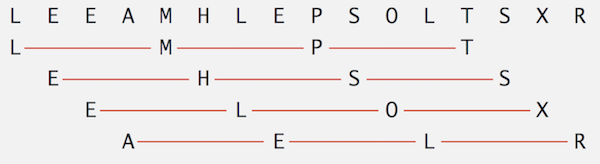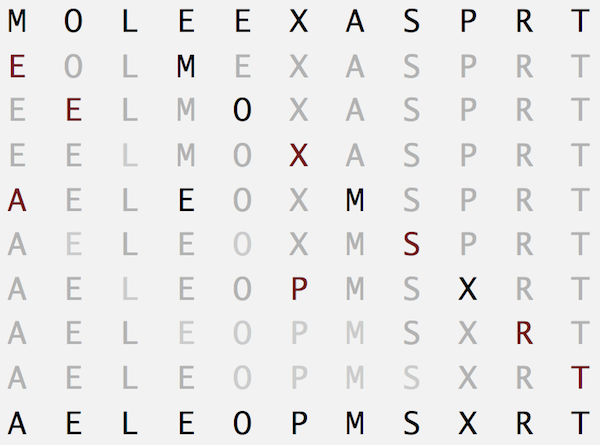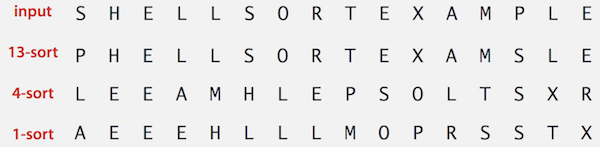Nothing special here. It’s just a blog post for summarising my algorithm learning course. Although some of them were already taught in the University, it’s still good to summarise here

# 1. Selection Sort

• In iteration i, find index min of smallest remaining entry.
• Swap a[i] and a[min]
function selectionSort(a: number[]): void {
const n = a.length;
for (let i = 0; i < n; i++) {

let min: number = i;
for (let j = i + 1; j < n; j++) {
if (a[j] < a[min]) {
min = j;
}
}

swap(a, i, min); // swap the 2 items
}
}

$"Complexity:"\phantom{\rule{0ex}{0ex}}\mathrm{O\left(}{N}^{2}\mathrm{/2\right)}~\mathrm{O\left(}{N}^{2}\right)$

# 2. Insertion Sort

• Like the reversed way of Selection Sort
• In iteration i, swap a[i] with each larger entry to its left.
function insertionSort(a: number[]): void {
const n = a.length;
for (let i = 0; i < n; i++) {
for (let j = i; j > 0; j--) {
if (a[j] < a[j-1]) {
swap(a, j, j-1);
} else {
break;
}
}
}
}

• Best case: If the array is in ascending order, insertion sort makes N - 1 compares and 0 exchanges.
• Eg: A E E L M O P R S T X
• Worst case: If the array is in descending order (and no duplicates), insertion sort makes ~ 1/2 N2 compares and ~ 1/2 N2 exchanges.
• Eg: X T S R P O M L E E A
• Still a bit better than Selection Sort

# 3. Shell Sort

## 3.1 h-Sorted Array

An h-sorted array is h interleaved sorted sub-sequences. Here is a 4-sorted array• To h-sort an array, use insertion sort with stride length h. Which means, for each iteration i, instead of going back by one step each, go back h steps. Here is an example of 3-sorting an array.## 3.2 What is Shell Sort?

• Move entries more than one position at a time by h-sorting the array
• h-sort array for decreasing sequence of values of h until we reach a 1-sorted array
• For example, 13-sort the array, 4-sort the result and then 1-sort the array to get the final sorted array• Some increasing of h values to use
• 3x + 1: 1, 4, 13, 40, 121, 364, …
• Sedgewick: 1, 5, 19, 41, 109, 209, 505, 929, 2161, 3905, …

## 3.3 Sample Code

class Shell {
public static void sort(Comparable[] a) {
int N = a.length;
int h = 1;

// 1, 4, 13, 40, 121, 364, ...
while (h < N / 3) h = 3 * h + 1;

// repeat until we get a 1-sorted array
while (h >= 1) {
// h-sort the array using insertion sort but with stride length h
for (int i = h; i < N; i++) {
for (int j = i; j >= h && less(a[j], a[j - h]); j -= h)
swap(a, j, j - h);
}
h = h / 3;
}
}
}